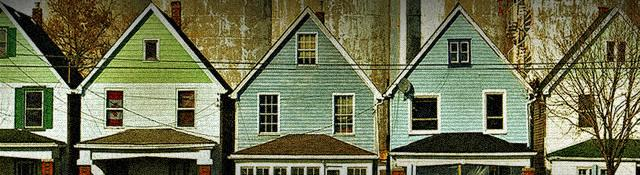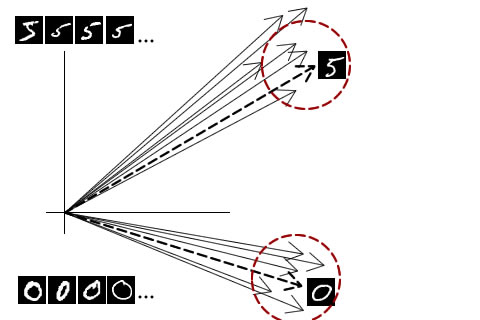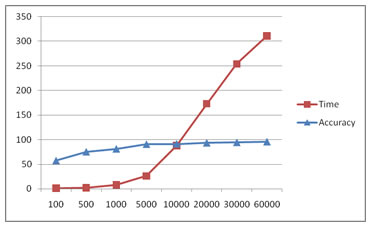## K-Nearest Neighbours: Classify (recognize) things

Recognizing things is a common problem in machine learning. Even in the SuDoKu Solver demo, we require a mechanism to identify digits in the SuDoKu grid. To do this, we'll use a simple algorithm called K-Nearest. It's one of the simplest machine learning algorithms. The results aren't going to be robust, but we'll get some good results.

## The K-Nearest Neighborhood Classification Algorithm

Like any machine learning algorithm, K-Nearest has two phases. In the first, you train the algorithm to recognize certain classes. In the second, you use all the training to classify test cases.

During the training phase, K-Nearest simply stores the training patterns you supply and their appropriate classification (which you supply).Suppose you want to train K-Nearest to identify the image as a '5' or a '0'. So you would supply as many images of '5' and '0' as you can. You also tell the algorithm which image is what. This is called the label for an image. You'd 'label' the first image above as a '5'. Similarly the second image as a '0', and so on.

After you're done training, you can have K-Nearest classify images for you. To classify an image, you must supply two things: the image to classify and a number k.

This k defines is the neighborhood in which training data is consulted. Consider this image:Here's what everything in the figure means:

• Thin solid arrows: These represent the training data. The arrows pointing to the top-right are for '5' and the ones pointing bottom-right are for '0'. These are just images converted into a vector.
• Thick dashed arrows: These represent the testing data. Again, these two arrows are just images converted into vectors.
• Red dashed circles: These are circles of radius 'k'.

Now here's how the k-nearest algorithm works. When given a vector, it checks all neighbors around it up till a distance of 'k'. Within these neighbors, someone one label will have the maximum number of vectors.

For example, consider the '0's dashed vector above. Every neighbor till a distance of 'k' has the label '0'. So the given vector must be a zero too. Simple as that.

Similarly for 5's dashed vector above. Neighbors till a distance of 'k' are all labelled as '5'. So the given vector is a 5.

## Deciding k in K-nearest neighbors

Deciding a "good" k for your data is very important. If you choose a big K, you might end up including unwanted vectors in the neighborhood. If your K is very small, you might not have enough vector to correctly "identify" a label.

So how do you find a good k? Well, you use heuristics and find, by hit and trial, a decent value.

## Disadvantages of K-Nearest neighbors

K-Nearest is the most simplest algorithm you can use for classifying things. It isn't optimized for speed or space.

You need lots of training samples to ensure lots of vectors are withing the 'k' sphere. And you need to have ALL training vectors in memory at all times. Then you compare a single test vector against LOTS of a training samples (to ensure they're within the 'k' sphere)/.

In short, its really slow and consumes a lot of memory.

## My results with K-Nearest Neighbors

I used the MNIST handwritten digits dataset. It has 60,000 training samples+labels and 10,000 test samples+labels. I varied the number of training samples given and checked the accuracy, memory used and time. Here are my results:

Training samples Time to test 1000 samples (sec) Memory used (KB) Accuracy (%)
100 1 s 4,328K 57.70 %
500 2 s 6,800K 75.50 %
1000 8 s 9,868K 81.50 %
5000 26 s 34,480 K 91.00 %
10000 88 s 65, 220 K 91.60 %
20000 173 s 128, 608 K 93.70 %
30000 254 s 188, 192 K 94.70 %
60000 311 s 372, 648 K 96.10 %

That looks exponential! Not good!Time complexity and increasing accuracy (Note that the horizontal axis is not linear)

## Summary

K-Nearest Neighbour is a very simple machine learning algorithm. Its simplicity makes it slow and is a memory eating monster :P

Utkarsh Sinha created AI Shack in 2010 and has since been working on computer vision and related fields. He is currently at Microsoft working on computer vision.